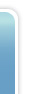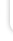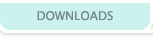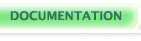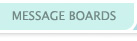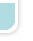## Math Functions

Trigonometric functions use a certain convention for angles that you may think a little odd. Zero degrees points due east and ninety degrees is due north. If you are using another convention in your game--such as zero degrees due north--then you will have to constantly convert between them when using the trig functions. I advise you not to use another convention in your game. Some things are worth fighting, but not this.

You will need to use Fixed-point numbers with these functions.

fcos(), fsin(), fatan2() and the like are for things that need more than 0 to 360 for angles. Verge defines a constant FIXED_PI, which is approximately the mathematical constant PI * 65536, which is used to measure the angles more accurately since we don't have floats. 2 * FIXED_PI is the same as 360 degrees.

• abs() Returns the absolute value of a number.
• acos() Returns the arccosine of a given value in fixed-point notation.
• asin() Returns the arcsine of the input value in fixed-point notation.
• atan() Returns the arctangent of the given value in fixed-point notation.
• atan2() Calculates arctan of y,x
• cbrt() Returns the cube root of a number.
• cos() Returns the cosine of the specified angle in fixed-point notation.
• facos() Same as acos but returns 16.16 fixed point radians, for precision.
• fasin() Same as asin, but returns 16.16 fixed point radians, for precision.
• fatan() Same as atan, but returns 16.16 fixed point radians, for precision.
• fatan2() Same as atan2, but returns 16.16 fixed point radians, for precision.
• fcos() Same as cos(), but takes a 16.16 fixed point radian angle.
• fsin() Same as sin(), but takes a 16.16 fixed point radian angle.
• ftan() Same as tan(), but takes a 16.16 fixed point radian angle.
• max() Returns the largest of two input numbers.
• min() Returns the smallest of two input numbers.
• pow() Raises base to exp power
• sgn() Returns if the number is positive, negative or zero.
• sin() Returns the sine of a given angle in fixed-point notation.
• sqrt() Returns the square root of the given number
• tan() Returns the tangent of the given angle in fixed-point notation.
• Talkback

Post a new comment?

Talkback #2 written by Omni on 2004-06-04.

Example code

```
int variable;

variable = Sin(180);

//Trig functions return results in 16.16 fixed point.

//Simple english:  multiplied by 65,536.

//Bitshift down by 16, or divide by 65,536 for fixed point

//to get the final result.

variable = variable >> 16;

int variablex, variabley;

variablex = Cos(180);

variabley = Sin(180);

int vectorlength;

vectorlength = 30;

//Do all math functions at 16.16 level,

//Then bitshift down by 16.

//If you bitshift the trig result first,

//your math will be very flawed.

//Do math at the fixed point level.

vectorx = (variablex * vectorlength) >> 16

vectory = (variabley * vectorlength) >> 16

```

Also, link for general purpose trig information, and basics:

Sin and Cos: The Programmer's Pals!

[edit: thanks grue. -omni]

Talkback #1 written by torin on 2004-06-03.

If you need trig functions that support fractional angles (35.62 degrees, for example), take a look at the Castle Heck raycaster demo. It demonstrates how to use lookup tables loaded from files for this purpose.
 Doc Nav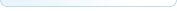Your docs View All Docs If you log in, you can edit the documentation, or create your own documents and tutorials!